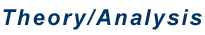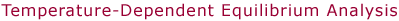This technique is used to determine enthalpy and entropy changes for equilibrium reactions of the type shown below.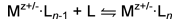Temperature-dependent equilibrium experiments have been used extensively in the Bowers group to obtain thermodynamic information for reactions of transition metal cations M+ with ligands L such as H2 and CH4. More recently, this method has been used to investigate hydration (L=H2O) of singly- and multiply-charged peptide cations and anions.     The equilibrium experiments are carried out in the Bowers group on the Quad-Cell-Quad and ESI instruments. The key component in each of these instruments is the drift cell where the equilibrium reaction takes place. The cell temperature can be precisely controlled such that experiments can be performed anywhere in a range from approximately 80 K (the temperature of liquid N2 at atmospheric pressure) up to as high as 800 K. The temperature within the drift cell is monitored using platinum resistors and/or thermocouple gauges which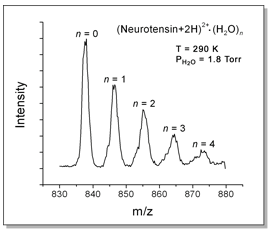are attached inside and outside the cell within the vacuum chamber. Multiple temperature readings are useful for detecting any temperature gradients across the cell. Cooling is accomplished using a controlled flow of dry nitrogen gas which has been chilled using liquid nitrogen. Heating is accomplished either by heating the flow of nitrogen gas using external heaters or by electrical heaters imbedded within the drift cell itself. With some care, temperatures can be kept within about ±0.5 K. The drift cell is filled with several Torr of the reactant gas L whose pressure is measured using a capacitance manometer (a Baratron unit, usually equipped with a 10 Torr head).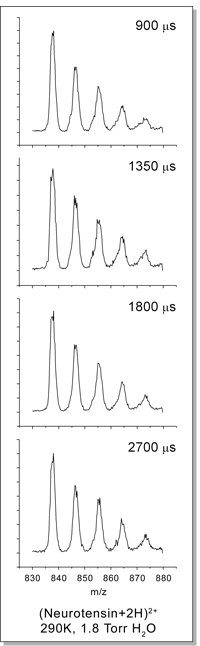After the ensuring that the temperature of the drift cell and its contents is stable, equilibrium measurements can be made. Mz+/- ions are injected into the cell containing the reactant gas L to form Mz+/-·Ln clusters . At the relatively high pressures of the cell, the ions are quickly thermalized by collisions with the neutral gas. The ions drift through the cell under the influence of a weak electric field which only marginally perturbs their thermal energies.      Ions exiting the drift cell are mass-analyzed with a quadrupole. An example of a resulting mass spectrum is shown above for the 13-amino-acid peptide neurotensin. The doubly-protonated ion binds up to 4 water molecules when it is exposed to 1.2 Torr of water vapor in the cell at 290 K.     The amount of time that the ions spend in the cell is directly proportional to the cell pressure and inversely proportional to the electric field (see Ion Mobility Theory). By adjusting the pressure and the voltage across the cell, the reaction time can be controlled. It is important to make certain that the drift time is long enough to ensure equilibrium of the reaction has been achieved. To do this, mass spectra of the ions exiting the drift cell are measured as a function of drift time. If the relative intensities of the peaks remain unchanged as drift time is increased, equilibrium conditions have been met. This is illustrated in the series of spectra shown at left. As drift time is increased from 900 to 2700 μs, the relative peak heights stay the same.     Since the intensities of the peaks in the mass spectra are proportional to the concentrations of the corresponding ions in the drift cell, the equilibrium constants K of the reactions can be determined using the equation below.In this expression, I(Mz+/-·Ln) is the product ion intensity, I(Mz+/-·Ln-1) is the reactant ion intensity, P0 is the standard-state pressure and PL is the pressure of the neutral reactant in the drift cell. The equilibrium constants can then be used to calculate standard-state Gibb's free energies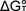using the expression below where R is the gas constant and T is the temperature.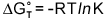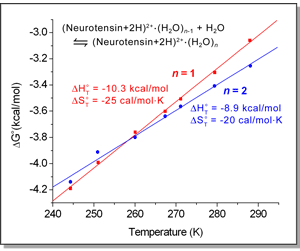Enthalpy and entropy changes,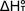and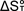, respectively, for the equilibrium reaction can be obtained by plottingversus T. This yields a straight line with an intercept equal toand a slope equal to -.*The values determined by this method are valid over the temperature range of the experiment. Bond dissociation energies at T = 0 K (-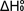) can obtained for simpler systems by fitting the experimental data using a statistical mechanical model based on vibrational frequencies and molecular geometries obtained, for example, from DFT calculations.   * Note: This is equivalent to the more usual van't Hoff plot of log(K) vs. 1/T.